# Measurement of Pulse and Transition Characteristics

This example shows how to analyze pulses and transitions and compute metrics including rise time, fall time, slew rate, overshoot, undershoot, pulse width, duty cycle, and pulse period.

### Clock Signal with Noise

First view the samples from a noisy clock signal.

```load clocksig clock1 time1 Fs plot(time1,clock1) xlabel('Time (seconds)') ylabel('Voltage')```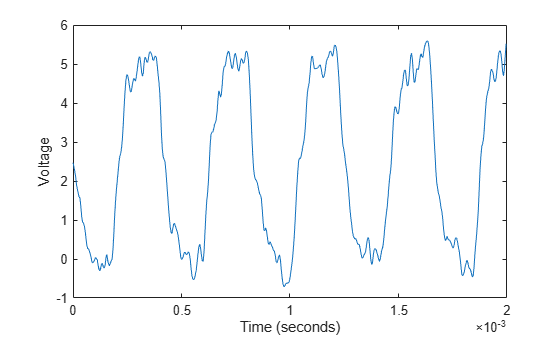### Estimate State Levels

Use `statelevels` with no output argument to visualize the state levels. The histogram method is used to estimate the state levels with these steps:

1. Determine the minimum and maximum amplitudes of the data.

2. For the specified number of histogram bins, determine the bin width, which is the ratio of the amplitude range to the number of bins. Use optional input arguments to specify the number of histogram bins and histogram bounds.

3. Sort the data values into the histogram bins.

4. Identify the lowest and highest indexed histogram bins with nonzero counts.

5. Divide the histogram into two subhistograms.

6. Compute the state levels by determining the mode or mean of the upper and lower histograms.

`statelevels(clock1)`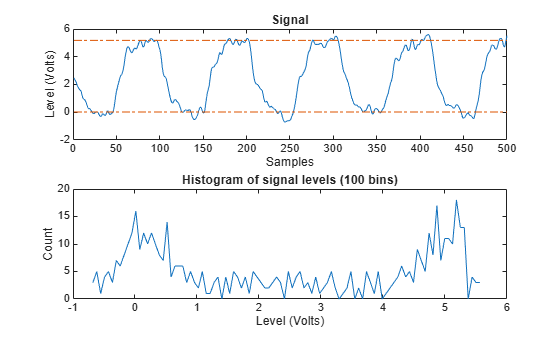```ans = 1×2 0.0138 5.1848 ```

The computed histogram is divided into two equal sized regions between the first and last bin. The mode of each region of the histogram is returned as an estimated state level value in the command window.

### Measure Rise Time, Fall Time and Slew Rate

Rise time is the duration between the instants where the rising transition of each pulse crosses from the lower to the upper reference levels. Fall time is the duration between the instants where the falling transition of each pulse crosses from the upper to the lower reference levels. The default reference levels for computing rise time and fall time are set at 10% and 90% of the waveform amplitude.

Use `risetime` with no output argument to visualize the rise time of positive-going edges. Then, use `falltime` with no output argument to visualize the fall time of negative-going edges. Specify reference levels as [`20` `80`] and state levels as [`0` `5`].

`risetime(clock1,time1)`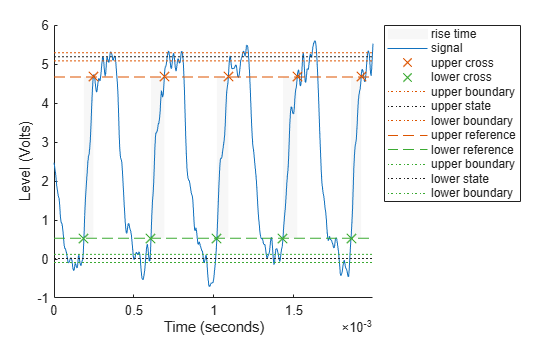```ans = 5×1 10-4 × 0.5919 0.8344 0.7185 0.8970 0.6366 ```
`falltime(clock1,time1,'PercentReferenceLevels',[20 80],'StateLevels',[0 5])`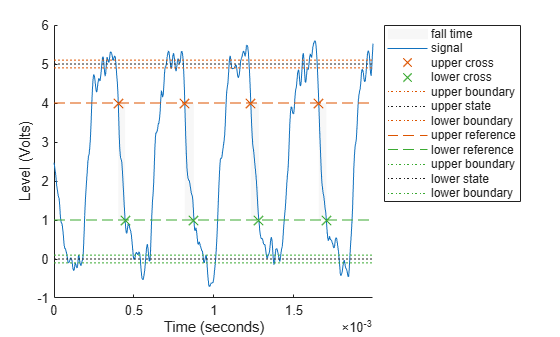```ans = 4×1 10-4 × 0.4294 0.5727 0.5032 0.4762 ```

Obtain measurements programmatically by calling functions with one or more output arguments. For uniformly sampled data, you can provide a sample rate in place of the time vector. Use `slewrate` to measure the slope of each positive-going or negative-going edge.

`sr = slewrate(clock1(1:100),Fs)`
```sr = 7.0840e+04 ```

### Analyze Overshoot and Undershoot

Now view data from a clock with significant overshoot and undershoot.

```load clocksig clock2 time2 Fs plot(time2,clock2) xlabel('Time (seconds)') ylabel('Voltage')```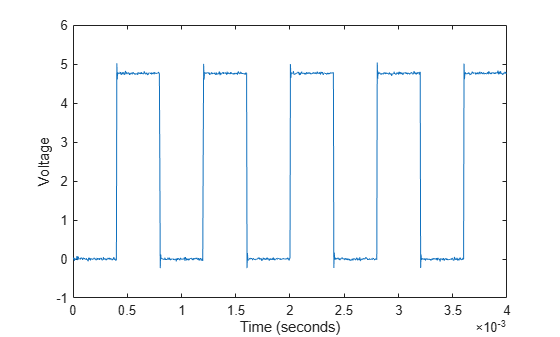Underdamped clock signals have overshoots. Overshoots are expressed as a percentage of the difference between state levels. Overshoots can occur just after an edge, at the start of the post-transition aberration region. Use the `overshoot` function to measure these postshoot overshoots.

`overshoot(clock2(95:270),Fs)`
```ans = 2×1 4.9451 2.5399 ```
`legend('Location','NorthEast')`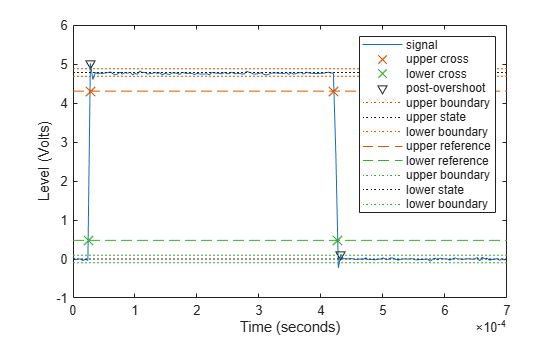Overshoots may also occur just before an edge, at the end of the pre-transition aberration region. These are called preshoot overshoots.

Similarly, you can measure undershoots in the pre- and post-aberration regions. Undershoots are also expressed as a percentage of the difference between the state levels. Use optional input arguments to specify the regions in which to measure aberrations.

`undershoot(clock2(95:270),Fs,'Region','Postshoot')`
```ans = 2×1 3.8499 4.9451 ```
`legend('Location','NorthEast')`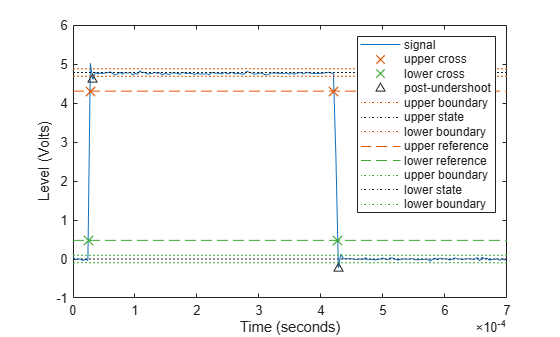### Measure Pulse Width and Duty Cycle

Width is the duration between the mid-reference level crossings of the first and second transitions of each pulse. Use `pulsewidth` with no output argument to plot highlighted pulse widths. Specify a positive polarity.

`pulsewidth(clock2, time2,'Polarity','Positive');`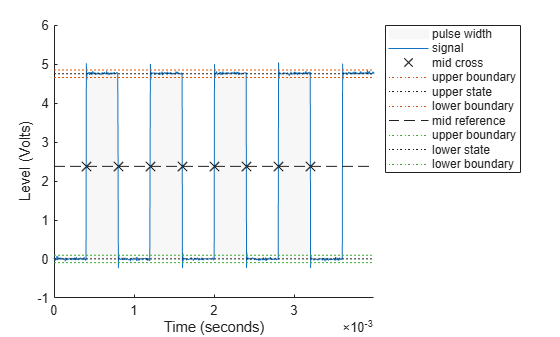Use `dutycycle` to compute the ratio of the pulse width to the pulse period for each negative-polarity pulse.

`d = dutycycle(clock2,time2,'Polarity','negative')`
```d = 3×1 0.4979 0.5000 0.5000 ```

Use `pulseperiod` to obtain the periods of each cycle of the waveform. The period is the duration between the first transition of the current pulse and the first transition of the next pulse. Use this information to compute other metrics like the average frequency of the waveform or the total observed jitter.

```pp = pulseperiod(clock2, time2); avgFreq = 1./mean(pp)```
```avgFreq = 1.2500e+03 ```
`totalJitter = std(pp)`
```totalJitter = 1.9866e-06 ```